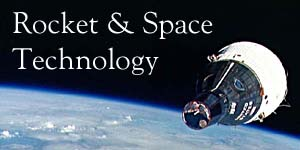BASICS OF SPACE FLIGHT

In this page we classify and describe the types of chemical propellants typically used in rocketry.  Included are tables listing the properties of the most frequently used fuels and oxidizers.

• Liquid Propellants
• Solid Propellants
• Hybrid Propellants
• Tables of Properties

In this page we describe how a rocket works by introducing and explaining the physics of rocket propulsion.  We will explain how a rocket engine accelerates the hot gases generated by the combustion of propellant to produce thrust.  Engine types and thrust chamber design equations are presented.  As we introduce the necessary formulae, example problems are provided to demonstrate their use.

• Thrust
• Conservation of Momentum
• Impulse & Momentum
• Combustion & Exhaust Velocity
• Specific Impulse
• Rocket Engines
• Power Cycles
• Engine Cooling
• Solid Rocket Motors
• Monopropellant Engines
• Staging

In this page we define an orbit and describe the various types.  We will introduce Newton's laws of motion and Universal Gravitation and explain how they apply to the motions of planets and satellites.  We'll examine how the Earth, Moon, Sun, and atmosphere affect a satellite's orbit, and we'll illustrate some common orbital maneuvers.  Many formulae will be introduced and demonstrated by way of example problems.

• Conic Sections
• Orbital Elements
• Types of Orbits
• Newton's Laws of Motion and Universal Gravitation
• Uniform Circular Motion
• Motions of Planets and Satellites
• Launch of a Space Vehicle
• Position in an Elliptical Orbit
• Orbit Perturbations
• Orbit Maneuvers
• The Hyperbolic Orbit

In this page we present the patched-conic method for approximating spacecraft trajectories between planets. We will define and solve the "Gauss problem" as it applies to interplanetary orbits, and we'll examine the trajectories a spacecraft flies while within the spheres of influence of its departure and arrival planets.

• Heliocentric Transfer Orbit
• The Gauss Problem
• Determining Orbital Elements
• Hyperbolic Departure and Approach
• Gravitational Assist

Appendices

The following are the appendices referenced in Parts I through IV above.